Test: Statistical Description Of Data - 3

# Test: Statistical Description Of Data - 3

Test Description

## 40 Questions MCQ Test Business Mathematics and Logical Reasoning & Statistics | Test: Statistical Description Of Data - 3

Test: Statistical Description Of Data - 3 for CA Foundation 2023 is part of Business Mathematics and Logical Reasoning & Statistics preparation. The Test: Statistical Description Of Data - 3 questions and answers have been prepared according to the CA Foundation exam syllabus.The Test: Statistical Description Of Data - 3 MCQs are made for CA Foundation 2023 Exam. Find important definitions, questions, notes, meanings, examples, exercises, MCQs and online tests for Test: Statistical Description Of Data - 3 below.
Solutions of Test: Statistical Description Of Data - 3 questions in English are available as part of our Business Mathematics and Logical Reasoning & Statistics for CA Foundation & Test: Statistical Description Of Data - 3 solutions in Hindi for Business Mathematics and Logical Reasoning & Statistics course. Download more important topics, notes, lectures and mock test series for CA Foundation Exam by signing up for free. Attempt Test: Statistical Description Of Data - 3 | 40 questions in 40 minutes | Mock test for CA Foundation preparation | Free important questions MCQ to study Business Mathematics and Logical Reasoning & Statistics for CA Foundation Exam | Download free PDF with solutions
 1 Crore+ students have signed up on EduRev. Have you?
Test: Statistical Description Of Data - 3 - Question 1

### The data given below refers to the marks gained by a group of students: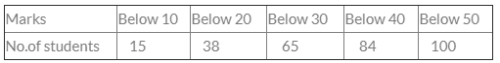Then the no. of students getting marks more than 30 would be ______________.

Test: Statistical Description Of Data - 3 - Question 2

### There were 200 employees in an office in which 150 were married. Total male employees were 160 out of which 120 were married. What was the number of female unmarried employees?

Test: Statistical Description Of Data - 3 - Question 3

### From which graphical representation, we can calculate partition values?

Test: Statistical Description Of Data - 3 - Question 4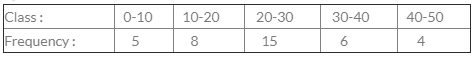​For the class 20-30, cumulative frequency is

Test: Statistical Description Of Data - 3 - Question 5

Difference between the maximum and minimum value of a given data is called

Test: Statistical Description Of Data - 3 - Question 6

The best method of presentation of data is

Test: Statistical Description Of Data - 3 - Question 7

A pie diagram is used to represent the following data: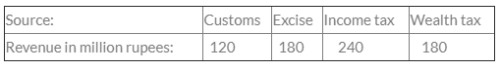The central angles in the pie diagram corresponding to income tax and wealth tax respectively:

Test: Statistical Description Of Data - 3 - Question 8

The following data relates to the marks of a group of  students.
Marks                         No. of Students
More than 70%                          07
More than 60%                          18
More than 50%                          40
More than 40%                          60
More than 30%                          75
More than 20%                         100
How many students have got marks less than 50%?

Detailed Solution for Test: Statistical Description Of Data - 3 - Question 8

Explanation : 40 students got marks more than 50%

Let Total students = 100

Students got marks less than 50% =  100 - 40

= 60 students.

Test: Statistical Description Of Data - 3 - Question 9

If calss interval is 10-14, 15-19, 20-24, then the first class is

Test: Statistical Description Of Data - 3 - Question 10

Which of the following is not a two dimensional diagram?

Test: Statistical Description Of Data - 3 - Question 11

The difference between the upper and lower limit of a class is called ___________.

Test: Statistical Description Of Data - 3 - Question 12

To draw Histogram, the frequency distribution should be:

Test: Statistical Description Of Data - 3 - Question 13

Cost of Sugar in a month under the heads raw materials, labour, direct production and others were 12, 20, 35 & 23 units respectively. The difference between their central angles for the largest & smallest components of the cost of Sugar is

Detailed Solution for Test: Statistical Description Of Data - 3 - Question 13

Since we have given that
12, 20, 35, 23 units.
Total units would be
12 + 20+ 35 + 23 = 90 Units
Difference between largest and smallest components is given by
35 - 12 = 23
So, the central angle for the difference would be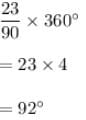Test: Statistical Description Of Data - 3 - Question 14

What is a exclusive series?

Test: Statistical Description Of Data - 3 - Question 15

The breadth of the rectangle is equal to the length of the class interval in

Test: Statistical Description Of Data - 3 - Question 16

The frequency of class 20-30 in the following data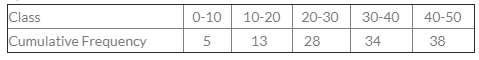Test: Statistical Description Of Data - 3 - Question 17

Frequency Density can be termed as:

Test: Statistical Description Of Data - 3 - Question 18

The Graphical representation by which median is calculated is called

Test: Statistical Description Of Data - 3 - Question 19

Arrange the following dimension wise : pie-diagram, bar-diagram and cubic diagram.

Test: Statistical Description Of Data - 3 - Question 20

For the overlapping classes 0-10, 10-20, 20-30 etc. The class mark of the class 0-10 is

Test: Statistical Description Of Data - 3 - Question 21

In Histogram, the classes are taken

Test: Statistical Description Of Data - 3 - Question 22

The curve obtained by joining the points, whose x-coordinates are the upper limits of the class-intervals and y coordinates are corresponding cumulative frequencies is called

Test: Statistical Description Of Data - 3 - Question 23

For non-overlapping classes 0-19, 20-39, 40-59 the class mark of the class 0-19 is

Test: Statistical Description Of Data - 3 - Question 24

The Chronological classification of data are classified on the basis of:

Test: Statistical Description Of Data - 3 - Question 25

An ogive can be prepared in ____________different ways.

Test: Statistical Description Of Data - 3 - Question 26

Diagrammatic representation of the cumulative frequency distribution is

Test: Statistical Description Of Data - 3 - Question 27

For overlapping class-intervals the class limit & class boundary are

Test: Statistical Description Of Data - 3 - Question 28

Classification is of

Detailed Solution for Test: Statistical Description Of Data - 3 - Question 28

Test: Statistical Description Of Data - 3 - Question 29

Upper boundary of any class coincides with the lower boundary of the next class.

Test: Statistical Description Of Data - 3 - Question 30

For obtaining frequency polygon we join the successive points whose abscissa represent the corresponding class frequency________

Test: Statistical Description Of Data - 3 - Question 31

Excepting the first and the last, all other class boundaries lie midway between the upper limit of a class and the lower limit of the next higher class.

Test: Statistical Description Of Data - 3 - Question 32

Difference between the maximum & minimum value of a given data is called

Test: Statistical Description Of Data - 3 - Question 33

When one end of a class is not specified, the class is called

Test: Statistical Description Of Data - 3 - Question 34

The lower extreme point of a class of called

Test: Statistical Description Of Data - 3 - Question 35

When the width of all classes is same, frequency polygon has not the same area as the Histogram.

Test: Statistical Description Of Data - 3 - Question 36

To find the mode graphically

Test: Statistical Description Of Data - 3 - Question 37

When all classes have equal width, the heights of the rectangles in Histogram will be numerically equal to the

Test: Statistical Description Of Data - 3 - Question 38

Histogram emphasizes the widths of rectangles between the class boundaries.

Test: Statistical Description Of Data - 3 - Question 39

The difference between the upper boundary and the lower boundary of a class is called

Detailed Solution for Test: Statistical Description Of Data - 3 - Question 39

Class size is the difference between the upper boundary and the lower boundary of the class.

Test: Statistical Description Of Data - 3 - Question 40

Which of the following can be determined by using the Ogive curve?

Detailed Solution for Test: Statistical Description Of Data - 3 - Question 40

Both median and quartiles can be determined from the Ogive curve.

## Business Mathematics and Logical Reasoning & Statistics

131 videos|149 docs|87 tests
 Use Code STAYHOME200 and get INR 200 additional OFF Use Coupon Code
Information about Test: Statistical Description Of Data - 3 Page
In this test you can find the Exam questions for Test: Statistical Description Of Data - 3 solved & explained in the simplest way possible. Besides giving Questions and answers for Test: Statistical Description Of Data - 3, EduRev gives you an ample number of Online tests for practice

## Business Mathematics and Logical Reasoning & Statistics

131 videos|149 docs|87 tests